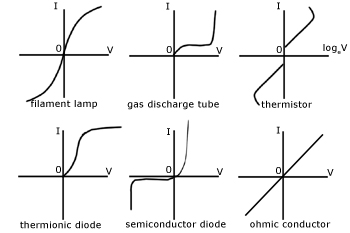# Ohms Law Question

So I hear people say Ohms law is v = IR is ohs law? But ohm means resistance so isn't V = IR just the specific formula to find voltage and ohs law is really V / IR and it can be split into three components (I mean the simple version not that giant circle with tons of formulas but the pyramid). The three components or formulas are:

V = IR
I = V/R
R = V/I

I am interested in finding the proper way to explain this to someone else because I get asked this a lot and that above is what I tell them? Thanks!

Ohm's Law actually says that the current between two points is proportional to the voltage across them. The proportionality is the resistance, and its unit is named in honour of Ohm.

The practical implication of that, is that given 2 you can find the other as you show with the three equations.

Rearranging one relationship into three different equations with each one being the subject of the equation does not make there be three versions of ohms law.

Ohms law is a description of a relationship not any one formula.

Basic algebra dictates that these are all the SAME.... V=IR, I=V/R, R=V/I and even your's, if you state it as V/IR=1 .

Using the principles of the math, you can figure out any missing piece if you have the other two. Since there are only three variables, there ultimately are only three things to solve and thus these three equations are already rearranged for you to solve the missing variable.

Good for solving power problems too.
Power (watts) = Current I (amps) x Voltage (volts), P=IV
Since V=IR, and I=V/R, can do some subbing to achieve
P = IV
or P = I x IR, aka (I^2)R, (sub for V)
or P = V/R x V, aka (V^2)/R (sub for I)
to find out how much power is dissipated in a transistor or instance.
BJT - you know V (Vce) and desired current I, so P = IV
MOSFET - you know Rds and desired current, so P = I^2R, and can calculate voltage drop = IxRds.

When I took Electronics in High School we were taught the [u]Ohm's Law Triangle[/u] to help us memorize the 3 variations.

At some point, one of my friends pointed out that it was "just algebra". I'm sure I knew a little algebra but for some reason I hadn't realized you could apply algebra to Ohm's Law!

Yup, that's all I did above.

When I was taught Ohm's Law at school, (55 years ago) it was stated as, "Provided the temperature of a given conductor is constant, the voltage developed between any two points is proportional to the current flowing between those points." There was no mention of resistance at all.

The modern version is, "The current through a conductor between two points is directly proportional to the potential difference across the two points." Again, no mention of resistance!

So, it doesn't really matter which of the three forms of the equation you use. They are none of them really Ohm's Law but are much more useful.

Russell.

Although these old descriptions describe a linear substance as being between the two points.
Ohm had no idea of non linear devices such as semiconductors where his law does not apply.

Hi,

Twinkle Twinkle Little Star, Power equals I squared R.
P=I2 R

Tom......(First coffee of the day..)

Tom, Tom, Tom, Tom, Tom

Twinkle, twinkle -

Night time here right now, so a proper end of discussion would be to point out, that Ohms law applies to both alternating current (AC) and direct current (DC) circuits & voltages.

@Gumpy_Mike :
What makes you think that Ohms law does not apply to semiconductors ?
By defintion, any current passing through a component, causing a voltage drop, will apply to the law.

Because ohms law states that the current through a device is proportional to the voltage across it. That does not apply to semiconductor junctions like diodes and LEDs.
If you think it does apply then go read some theory.

Anders53:
What makes you think that Ohms law does not apply to semiconductors ?

Have a look at this:(Source: http://www.a-levelphysicstutor.com)

TomGeorge:
Twinkle Twinkle Little Star, Power equals I squared R.
P=I2 R

Nothing to do with Ohm's Law. It's more closely related to Joule's Law.

Russell.

Ohm's law doesn't apply to nuclear IC's. Cole's law goes better with fission chips.

But that ratio -is- the resistance.

russellz:
When I was taught Ohm's Law at school, (55 years ago) it was stated as, "Provided the temperature of a given conductor is constant, the voltage developed between any two points is proportional to the current flowing between those points." There was no mention of resistance at all.

The modern version is, "The current through a conductor between two points is directly proportional to the potential difference across the two points." Again, no mention of resistance!

So, it doesn't really matter which of the three forms of the equation you use. They are none of them really Ohm's Law but are much more useful.

Russell.

polymorph:
But that ratio -is- the resistance.

You know that and I know that but George Ohm didn't define it.

Russell.

Fortunately, someone did.

Still wondering why a LED can be lit using a series resistor.
That dumb LED does not comply to Ohms law, but its lighting up anyway.
Gotta teach them LEDs a lesson, now I've been told better ...